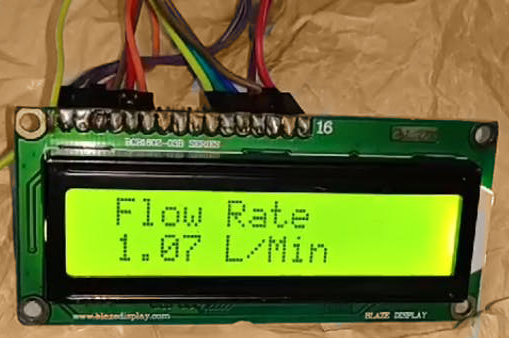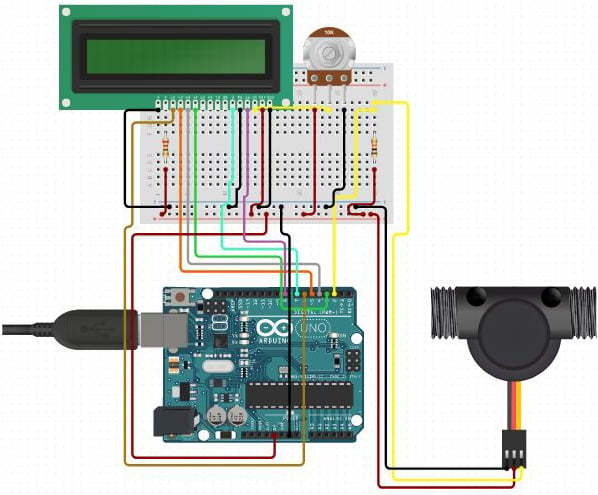Arduino

# Measuring water Flow Rate using Arduino and Flow SensorMarch 11, 2020 by rasika Joshi

ShareFlow sensor helps you to calculate the flow rate and volume of liquid that has passed through the pipe.

## Hardware

 1 Arduino Uno 1 LCD 16 X 2 1 Water flow sensor 1 connecting wires 1 Pipe

Flow sensor helps you to calculate the flow rate and volume of liquid that has passed through the pipe.

YFS201 Water Flow Sensor

The Water Flow sensor has 3 different wires RED, YELLOW, and BLACK as shown in the figure below. The red wire is generally utilized for supply voltage which ranges from 5V to 18V and the black wire is attached to GND. The yellow wire is utilized for the output, which can be read by an MCU.

The water flow sensor contains a pinwheel sensor that estimates the quantity of liquid that has passed through it.

The water flow sensor basically operates on the principle of hall effect. The water flow sensor is combined with a magnetic hall effect sensor, which produces an electric pulse with every strike.

Working of Project

In this project, we attached the water flow sensor to a pipe. If the output valve of the pipe is terminated, the output of the water flow sensor is zero that means no pulses.

And If the output valve of the pipe is opened. The water runs via the sensor, which in turn revolves around the wheel inside the sensor. In this situation, we can see pulses, which are produced from the sensor.

These pulses basically will work as an interrupt signal to the Arduino UNO.

The current time and loop time variable assure that for every one second the value of the flow_frequency is utilized for calculation of flow rate and volume. Once the calculation is completed, the flow_frequency variable is set to zero and the complete procedure is originated from the beginning.``````volatile int flow_frequency; // Measures flow sensor pulses
// Calculated litres/hour
float vol = 0.0,l_minute;
unsigned char flowsensor = 2; // Sensor Input
unsigned long currentTime;
unsigned long cloopTime;
#include <LiquidCrystal.h>
LiquidCrystal lcd(12, 11, 5, 4, 3, 9);
void flow () // Interrupt function
{
flow_frequency++;
}
void setup()
{
pinMode(flowsensor, INPUT);
digitalWrite(flowsensor, HIGH); // Optional Internal Pull-Up
Serial.begin(9600);
lcd.begin(16, 2);
attachInterrupt(digitalPinToInterrupt(flowsensor), flow, RISING); // Setup Interrupt
lcd.clear();
lcd.setCursor(0,0);
lcd.print("Water Flow Meter");
lcd.setCursor(0,1);
lcd.print("Circuit Digest");
currentTime = millis();
cloopTime = currentTime;
}
void loop ()
{
currentTime = millis();
// Every second, calculate and print litres/hour
if(currentTime >= (cloopTime + 1000))
{
cloopTime = currentTime; // Updates cloopTime
if(flow_frequency != 0){
// Pulse frequency (Hz) = 7.5Q, Q is flow rate in L/min.
l_minute = (flow_frequency / 7.5); // (Pulse frequency x 60 min) / 7.5Q = flowrate in L/hour
lcd.clear();
lcd.setCursor(0,0);
lcd.print("Rate: ");
lcd.print(l_minute);
lcd.print(" L/M");
l_minute = l_minute/60;
lcd.setCursor(0,1);
vol = vol +l_minute;
lcd.print("Vol:");
lcd.print(vol);
lcd.print(" L");
flow_frequency = 0; // Reset Counter
Serial.print(l_minute, DEC); // Print litres/hour
Serial.println(" L/Sec");
}
else {
Serial.println(" flow rate = 0 ");
lcd.clear();
lcd.setCursor(0,0);
lcd.print("Rate: ");
lcd.print( flow_frequency );
lcd.print(" L/M");
lcd.setCursor(0,1);
lcd.print("Vol:");
lcd.print(vol);
lcd.print(" L");
}
}
}``````